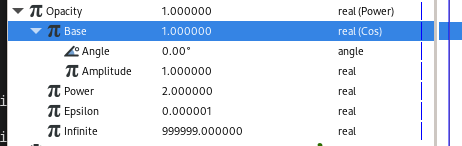# If else expression

HI.i want to reach this feature like if(x==0)x++;if(x>10)x=0;
I know the switch can get the expression of if(x>10)x=0 but how to get expression if(x==0)x++ .
My goal is moving a ball for step of 1 unit.if the ball get the point x==10,the ball will go back to original point x=0 and repeat the cycle previous.

Look like this gif show.but i don’t know how to do this in synfig.

if(@Frame%24==0)@P.y+=1;
if(@P.y>5)@P.y=0;

How about using constant type waypoints with a time loop?
digitalMotion.sifz (1.9 KB)

1 Like

Thanks for your sharing.it is a efficient method to get this kind of effect.
I just wonder how to get this effect automaticly.

You can do the animation automatically but there isn’t any advantage, but will only increase in complexity. You can also do cool stuff if you use math and exporters properly

But for your example it’s best what @GregorysonofCarl has suggested, to use time loop layer.

You can achieve something with converter Linear, which will increase periodically in linear way. Try changing the values and you will figure it out.

If you have to repeat some action you have to use Time Loop layer/converter doing this on your own is not useful at all.

There is also the step convert type which also creates stepped motion.

Thanks for inro.i will take a try.

I will take a look at that. thanks again.

What we really need us a “modulo” division conversion.

I’ve worked out a way of connecting a linear conversion, through a scale conversion, and a sin and cosine conversion, and then a vector angle conversion, which allow what I think this fellow is after, you can go round around a circle 360, 720, 1080 degrees linear, but through the conversions finally “vector angle” ( or arctan2 can also work) makes this go from 0 to 360 over and over. It’s bizarre but it functionally works like a modular division function.

So you could build one of these gadgets with a divide by 36 and it could be bribed to count from 0 to 9 over and over then you run that into the selected field of a switch function or something. I really need to find the file server so I can share files with you guys. There are a lot of really cool things you can do with conversions.

1 Like

You can upload files here in the forum.
You could also add you explanations to the documentation here: GitHub - synfig/synfig-docs: Synfig User Manual
In any case you could upload a screenshot of the parameters panel like I did in this thread: "Filling up" a shapeHey, thank you rodolforg! I spent a couple of hours today recreating this monstrosity, left unfinished, but you can count “one,two,three,and-uh” as we step 45 degrees at a time round the circle. But I’d much rather use a modulo conversion.
modulo.sifz (10.6 KB)
I’m instead working on some material that should help users lipsyncing with rhubarb.

1 Like

rodolfo, we also need the missing mate of conversions between types angle and real. You cant even hook the output of cosine to the input of another cosine to simulate cosine^2 because it accepts angles, outputs reals, and there is no real-to-angle converter. (I mean, there are other ways to skin a cat, but this one ought to be available too)Wouldn’t it work as shown below (`Pow` and `Cos`)?